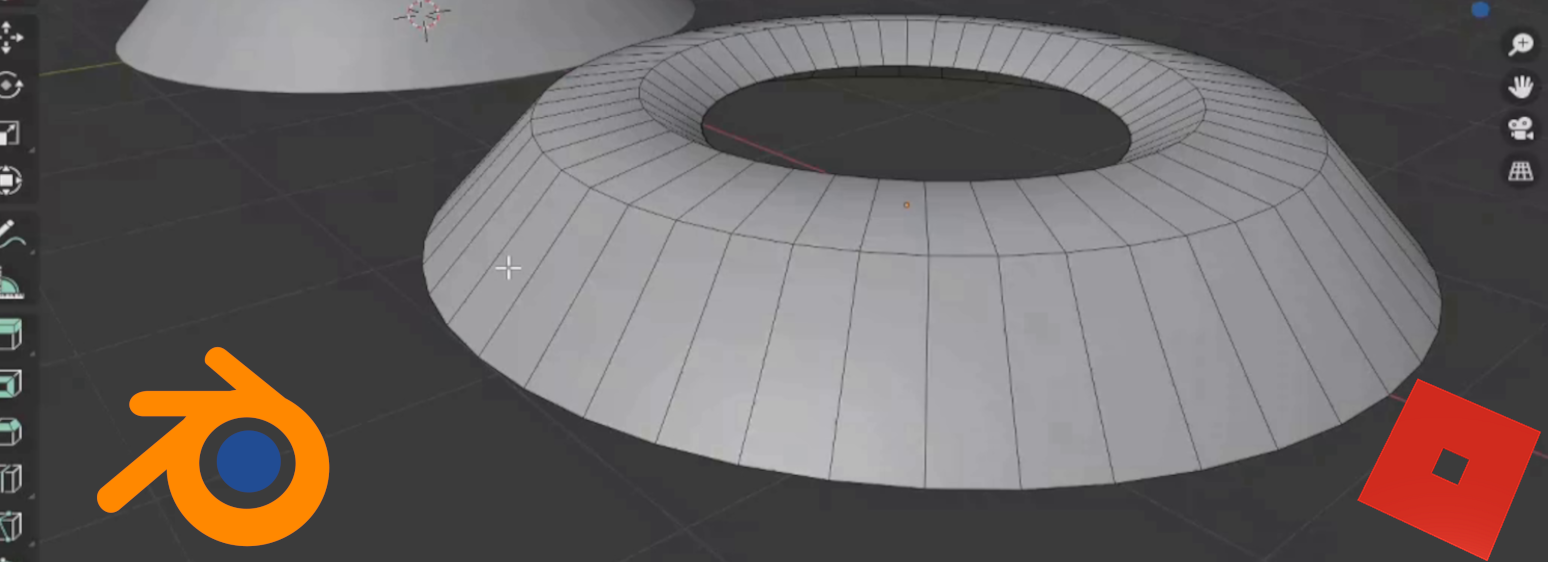# Reducing Geometry in Blender ModelsSome game engines can only take models below 10,000 polygons, making the process of reducing the geometry in a blender model essential to learn. In this post, we’re going to learn about reducing our polygon count to move a blender model into a game engine, in our case Roblox. We’re going to explore two ways of doing this, using the decimate modifier, and manually targeting specific geometry to reduce.

The setup

We’ve started by creating an object using a circle curve and then beveling it with a bezier curve. The resulting geometry is 1152 polygons. We then duplicated it twice. We’ll leave one alone, decimate one, and manually reduce the geometry of one.

Using Decimate

Starting with the decimate modifier, we place the modifier and slide the ratio to a point where the object still retains its shape, but has a reduced polygon count.

If you pull the ratio slider down too far, we can really deform the geometry so we need to balance that deformation with the reduced polygon count.

When we’re reducing geometry we take into account the engine we’re using, how close the object will be viewed from in game, and what the overall resolution of the game is. In our case we’re importing into roblox, and want a moderate amount of smoothness on the object.  So where we have it is good. If we apply smoothing to this we would be able to see, relatively speaking, what it will look like in Roblox.

Looking at this object in edit mode, we can see the geometry is haphazard. There are some triangles, there are some quads, there are some other polygons.

To understand this part of the process, and why decimate isn’t great for game engines, let’s understand how some engines process polygons and what effect this mixed bag has on rendering speed.

All engines, in the end result, process our polygons down to triangles. So we typically want to get as close to a triangle as possible without actually reducing the geometry to a triangle. this would be a quad. If we go higher than a quad, there will be a lot of processing on a shape to get it to a triangle, adding to the render time.

Why wouldn’t we want to reduce our geometry to triangles?  Because some engines will turn a triangle into three quads first, so the final render is down to two triangles.  We end up adding rendering time when we are trying to speed it up.  So generally we want to work in quads.

Manually reducing geometry

Let’s look at our last object. It’s already quads, so that’s a good start.

What we want to do is give our outer edges some solid geometry first. If we don’t do this, rendering engines and modifiers might deform these edges. We would take this same approach if we had sharp edges, or any other geometry we want to ensure is solid and stable. Here we slide the edges above our outer edges down, using gg, to give the end edges more support.

Now to reduce the rest of the geometry.

When we look at the shape of our object, we want to mentally reduce it to its base form(s). Then we can disolve any geometry that isn’t strictly needed. In this case I can see the final shape being a rhombus. That would give us our general shape, similar to the original.

We can then smooth the shape to bring it to the same look as the original.

And in these two cases, we’ve significantly reduced our polygon count.  In this last case, our polygons were reduced from 1152 to 384!

When we move these objects into Blender, we can see that the object on the left, our manually reduced object, our item on the right, our decimate object, and the original center object look very close to one another.

The difference now being that the modified objects will render much faster.

Wrap up

When working with models in Blender, and looking to move those to a game engine, we need to keep in mind the effect that lots of objects will have on the render time of our game. So reducing our geometry becomes an important part of the design and development process. Knowing that we can quickly use decimate, or take the time to manually reduce our geometry, and the why, when and how of each is very important to any game developer or asset designer.

I hope this post helps to understand a bit more why we need to reduce geometry, and two ways of doing this.

You can find this post as a video walkthrough on our Youtube page at Reducing Poly Count in Blender Models